Chapter 12

Similar to the electric charge, the magnetic charge conservation law is as follows: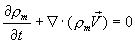(12.1)

In which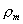is the magnetic charge density.

Let us prove that the magnetic charge conservation equation is valid for the electron.

Combine the electron velocity equation (10.11) and the magnetic field equation (5.2), then we get: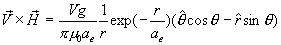(12.2)

As we know: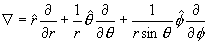(12.3)

Thus: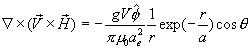(12.4)

From the magnetic charge density equation (3.1), thus:(12.5)

From equation (5.2) we know that: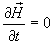(12.6)

Thus, we can get: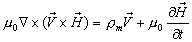(12.7)

Compare with the hydromagnetic equation (dynamo equation), which is as follows: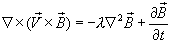(12.8)

We can see there is much similarity between these two equations. We then call the equation (12.7) as the hydromagnetic equation of electromagnetic field.

Because: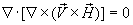(12.9)

Thus:(12.10)

Equation (12.10) is the electron magnetic charge conservation equation, which is also called the magnetic charge continuity equation.